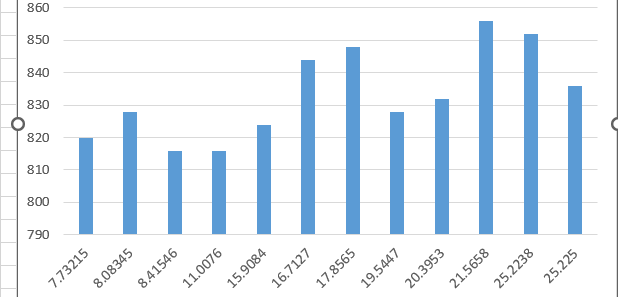# Problem about uniform distribution energy spectrum

Hello,i want to generate a sort of particle with a uniform distribution ,which energy is from 0-30MeV.
Here is my code,but the resuilt seems not right.Some energy didn’t appeared like 2MeV,3MeV etc.
Can you check my code and give some advice?

G4int a=0;
G4int b=30;
G4cout << “suijishu” << ( (rand() % (b-a+1))+ a)<<G4endl;

``````    fParticleGun->SetParticleEnergy((rand() % (b-a+1))+ a*MeV);
``````
1. Don’t use `rand()`. Use `G4UniformRand()`, which returns a floating point value between 0 and 1. Geant4 includes much better random engines and the Unix system does.

2. Your expression is only multiplying `a` by your units; the random value isn’t being multiplied. So there’s no guarantee the final value is physically meaningful.

3. Don’t use integers. Energy is a floating point value.

4. Specify your enengy range with energy units, then do a simple calculation.

``````G4double Emin = 0.*MeV;
G4double Emax = 30.*MeV;
G4double Ebeam = Emin + G4UniformRand()*(Emax-Emin);
fParticleGun->SetParticleEnergy(Ebeam);
``````

hello,i used your code and saw 1000 run results, the same question:only create some enenrgy,many energy values didn’t appear.

What is your histogram binning? Why do you think that 1000 events would fully populate 64-bit floating point values?

ok，here’s the histo.X is the energy value which created.and you see the problem.Just some value .
And plus,I run 100 times and emit 100 particles each run.
I thick maybe some wrong configuration about random value .Y is the frequency of the value occured.

So what is the mean and RMS of the number of entries for that particular binning? With 12 bins, you should have an average of 833 entries per bin. That’s what it looks like to me. Maybe make a plot of the number of entries per bin, see what that looks like?

You could do a sophisticated test, like a Kolomogorov-Smirnov comparison to a flat distribution, and see what P value comes out. I think you’re going to find that you’re getting exactly what you should, with normal statistical fluctuations.

emmm, why only 12 value created?I don’t know too much about how pseudo-random number product

There aren’t “12 values created.” You chose to create a histogram with only 12 bins. Therefore, there are only 12 bins filled with the full distribution of floating point values.

Why don’t you create a histogram with 8 bins, or 200 bins, or something else?